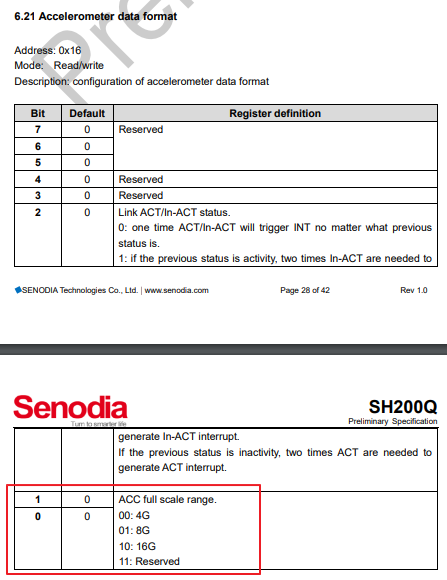# StickC - SH200Q Acceleration Range

• How to init the acceleration range to 16g for Sh200q on StickC with the MicroPython code?

• @zhufu86

I tried the code blow. But I don't know whether it is correct.

``````from hardware import sh200q
imu = sh200q.Sh200q(accel_fs=sh200q.ACCEL_FS_SEL_16G)
``````

• imu = sh200q.Sh200q(accel_fs=sh200q.ACCEL_FS_SEL_16G)

I tried the codes in the StickC and got the acceleration value four times as big as the value before. So I suppose SH200Q is initiated with 4g range if lack of accel_fs setting.
For temporary solution I devide the returned acceleration value by 4.

``````  x = imu.acceleration/4
y = imu.acceleration/4
z = imu.acceleration/4
``````

I also did some tests as below. No clue...

``````>>> imu = sh200q.Sh200q()
>>> imu._accel_so
8192
>>> imu.acceleration
0.995
>>> imu = sh200q.Sh200q(accel_fs=sh200q.ACCEL_FS_SEL_16G)
>>> imu._accel_so
2048
>>> imu.acceleration
4.058
>>> imu.acceleration/4
0.9985
>>> imu._accel_so=8192
>>> imu.acceleration
0.999
>>>
``````

• imu._accel_so=8192

Update...
No matter how I initiate SH200Q, I can not get the range larger than 4g.
Need more investigation.

• @zhufu86

It seems I need to write the correct configuration into the SH200Q register manually.Here is how to....

``````MicroPython ESP32_LoBo_v3.2.24 - 2018-09-06 on M5Stack with ESP32
>>> from hardware import sh200q
>>> imu = sh200q.Sh200q(accel_fs=sh200q.ACCEL_FS_SEL_16G)
>>> imu.acceleration
4.332
b'\x10'
>>> imu.i2c.writeto_mem(108,0x16,'\x12')
1
>>> imu.acceleration
1.086
>>>
``````

Is it a bug in module "hardware.sh200q"?

• ``````@property
def acceleration(self):
so = self._accel_so
data = self._regThreeShort(0x00)
return tuple([round(value / so, 3) for value in data])
``````

output data is not raw data, and ACCEL_FS_SEL_16G only measuring range, does not affect the value of acceleration

• I know, it a bug..., will fix it